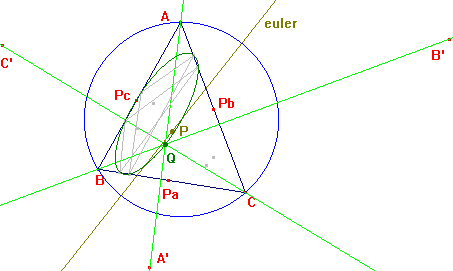r407

Let P be a point in the Euler line, Pa Pb Pc its cevian feet, A' B' C' the inverses of Pa Pb Pc in the circumcircle. Then AA', BB', CC' concur at a point Q in the conic that circumscribes the cevian triangles of the isogonals of X(115) and X(125).
When P = O is Q = O; when P = H, Q = X(24); when P = G, Q = K.